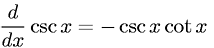Equations > Calculus > Differentiation/Differentals > Derivative of Cosecant

### Derivative of CosecantLatex Code:

MathML Code:

 $\fracd\mathrm{dx}\mathrm{csc}x=-\mathrm{csc}x\mathrm{cot}x$

MathType 5.0: